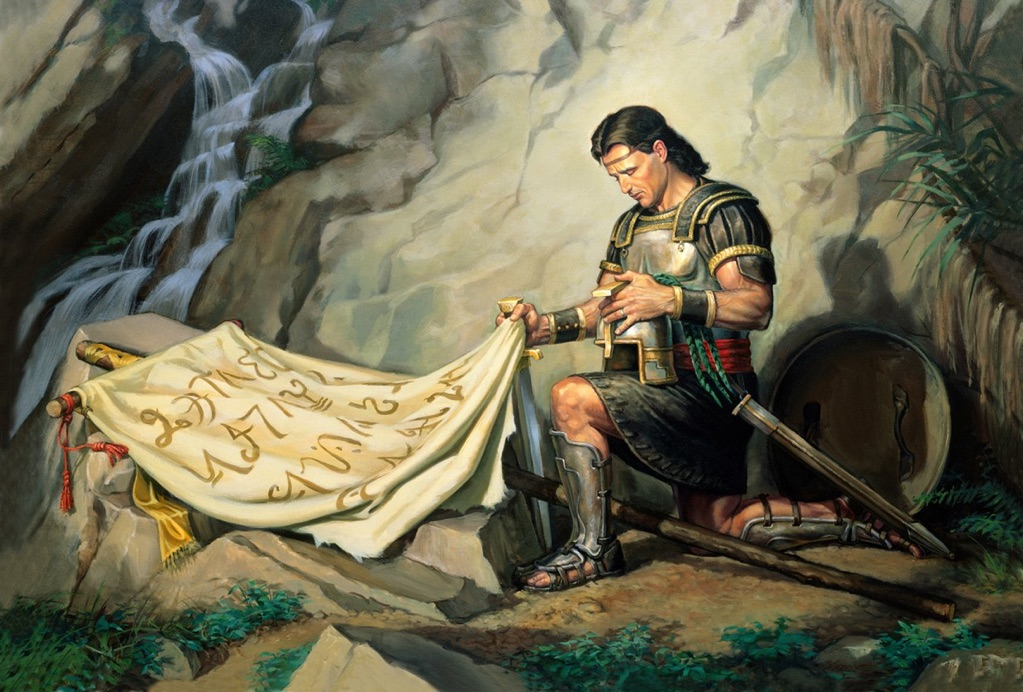M   E   M   B   E   R     —     N   E   W      Y   O   R   K      S   O   C   I   E   T   Y      O   F      I   L   L   U   S   T   R   A   T   O   R   S      •       N   A   T   I   O   N   A   L   L   Y      H   O   N   O   R   E   D